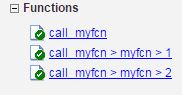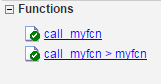# coder.ignoreConst

Prevent use of constant value of expression for function specializations

## Syntax

``coder.ignoreConst(expression)``

## Description

example

````coder.ignoreConst(expression)` prevents the code generator from using the constant value of `expression` to create function specializations. `coder.ignoreConst(expression)` returns the value of `expression`.```

## Examples

collapse all

Use `coder.ignoreConst` to prevent function specializations for a function that is called with constant values.

Write the function `call_myfn`, which calls `myfcn`.

```function [x, y] = call_myfcn(n) %#codegen x = myfcn(n, 'mode1'); y = myfcn(n, 'mode2'); end function y = myfcn(n,mode) coder.inline('never'); if strcmp(mode,'mode1') y = n; else y = -n; end end```

Generate standalone C code. For example, generate a static library. Enable the code generation report.

`codegen -config:lib call_myfcn -args {1} -report`

In the code generation report, you see two function specializations for `call_myfcn`.The code generator creates `call_myfcn>myfcn>1` for `mode` with a value of `'mode1'`. It creates `call_myfcn>myfcn>2` for `mode` with a value of `'mode2'`.

In the generated C code, you see the specializations `my_fcn` and `b_my_fcn`.

```static double b_myfcn(double n) { return -n; } static double myfcn(double n) { return n; } ```

To prevent the function specializations, instruct the code generator to ignore that values of the `mode` argument are constant.

```function [x, y] = call_myfcn(n) %#codegen x = myfcn(n, coder.ignoreConst('mode1')); y = myfcn(n, coder.ignoreConst('mode2')); end function y = myfcn(n,mode) coder.inline('never'); if strcmp(mode,'mode1') y = n; else y = -n; end end```

Generate the C code.

`codegen -config:lib call_myfcn -args {1} -report`

In the code generation report, you do not see multiple function specializations.In the generated C code, you see one function for `my_fcn`.

## Input Arguments

collapse all

Expression whose value is to be treated as a nonconstant, specified as a MATLAB expression.

collapse all

### Function Specialization

Version of a function in which an input type, size, complexity, or value is customized for a particular invocation of the function.

Function specialization produces efficient C code at the expense of code duplication. The code generation report shows all MATLAB function specializations that the code generator creates. However, the specializations might not appear in the generated C/C++ code due to later transformations or optimizations.

## Tips

• For some recursive function calls, you can use `coder.ignoreConst` to force run-time recursion. See Force Code Generator to Use Run-Time Recursion.

• `coder.ignoreConst(expression)` prevents the code generator from using the constant value of `expression` to create function specializations. It does not prevent other uses of the constant value during code generation.